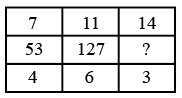Question 4

# Study the given pattern carefully and select the number that can replace the question mark (?) in it.Solution

$$(7)^2$$ + 4 = 49 + 4 = 53
$$(11)^2$$ + 6 = 121 + 6 = 127
$$(14)^2$$ + 3 = 196 + 3 = 199
$$\therefore$$ The correct answer is option C.

• Free SSC Study Material - 18000 Questions
• 230+ SSC previous papers with solutions PDF